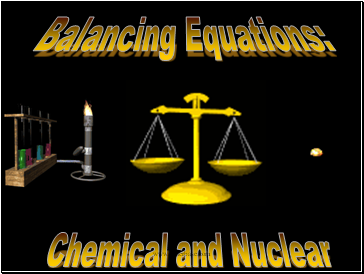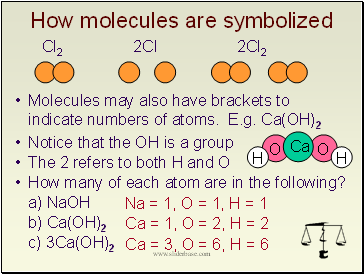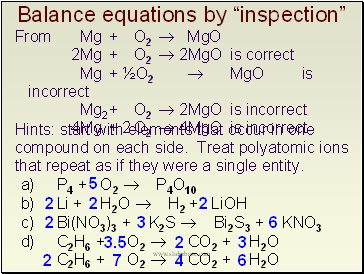# Chemical and NuclearPage 1

#### WATCH ALL SLIDES

Slide 1Balancing Equations:

Chemical and Nuclear

Slide 2## How molecules are symbolized

Cl2 2Cl 2Cl2

Molecules may also have brackets to indicate numbers of atoms. E.g. Ca(OH)2

Notice that the OH is a group

The 2 refers to both H and O

How many of each atom are in the following?

a) NaOH

b) Ca(OH)2

c) 3Ca(OH)2

Na = 1, O = 1, H = 1

Ca = 1, O = 2, H = 2

Ca = 3, O = 6, H = 6

Slide 3## Balancing equations: MgO

The law of conservation of mass states that matter can neither be created or destroyed

Thus, atoms are neither created or destroyed, only rearranged in a chemical reaction

Thus, the number of a particular atom is the same on both sides of a chemical equation

Example: Magnesium + Oxygen (from lab)

Mg + O2  MgO

However, this is not balanced

Left: Mg = 1, O = 2

Right: Mg = 1, O = 1

Slide 4## Balance equations by “inspection”

Hints: start with elements that occur in one compound on each side. Treat polyatomic ions that repeat as if they were a single entity.

5

2

3

3.5

2

7

4

6

2

2

2

2

6

3

C2H6 + O2  CO2 + H2O

a) P4 + O2  P4O10

b) Li + H2O  H2 + LiOH

c) Bi(NO3)3 + K2S  Bi2S3 + KNO3

d) C2H6 + O2  CO2 + H2O

From Mg + O2  MgO

2Mg + O2  2MgO is correct

Mg + ½O2  MgO is incorrect

Mg2 + O2  2MgO is incorrect

4Mg + 2 O2  4MgO is incorrect

Slide 5## Balance these skeleton equations:

a) Mg + 2HCl  MgCl2 + H2

b) 3Ca + N2  Ca3N2

c) NH4NO3  N2O + 2H2O

d) 2BiCl3 + 3H2S  Bi2S3 + 6HCl

e) 2C4H10 + 13O2  8CO2 + 10H2O

f) 6O2 + C6H12O6  6CO2 + 6H2O

g) 3NO2 + H2O  2HNO3 + NO

h) Cr2(SO4)3+ 6NaOH  2Cr(OH)3+ 3Na2SO4

i) Al4C3 + 12H2O  3CH4 + 4Al(OH)3

Slide 6## Returning to reaction types

We have looked at several types of reactions without worrying about balancing

However, all equations should be balanced

Predict the products and balance these:

(recall, metals above replace metals below, reactions with water yield metal hydroxides)

Cu + Fe2(SO4)3

NR (no reaction)

Zn + Li2CO3

Cu + AlCl3

Fe + CuSO4 

LiOH + H2

Al2O3

2

Ni + NaCl 

Al + CuCl2 

Go to page:
1  2